### IMO Shortlist 1985 problem 2

Kvaliteta:
Avg: 0,0
Težina:
Avg: 0,0
A polyhedron has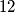$12$ faces and is such that:

(i) all faces are isosceles triangles,
(ii) all edges have length either$x$ or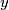$y$,
(iii) at each vertex either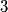$3$ or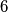$6$ edges meet, and
(iv) all dihedral angles are equal.

Find the ratio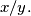$x/y.$
Izvor: Međunarodna matematička olimpijada, shortlist 1985